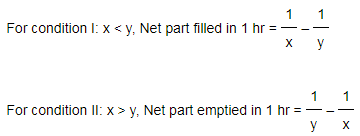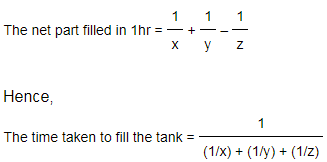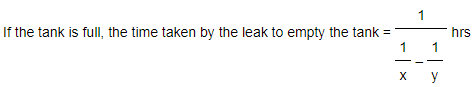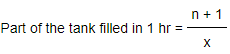# Pipes And Cisterns

#### Video Lesson on Pipes And Cisterns

Problems related to pipes & cisterns are similar to those of time and work but with a slight difference of conceptual agents like inlets & outlets.

Questions on pipes and cisterns can be mixed with questions on mixtures and alligations to increase the difficulty level of questions. Quantitative aptitude questions on pipes and cisterns, when mixed with ratios, can be a bit tricky. But a strong understanding of the concept behind solving them and practising as many questions as possible can help you easily solve them.

### Basic Terms:

Inlet: A pipe which is used to fill up the tank,cistern or reservoir is known as 'Inlet'. This type of nature indicates 'plus' or 'positive type' of work done.
Outlet: A pipe which is used to empty the tank, cistern or reservoir is known as 'Outlet'. This type of nature indicates 'minus' or 'negative type' type of work done.

#### Frequently asked aptitude questions on pipes and cisterns include:

1. Given time taken by individual pipes to empty/fill up a tank. How much does it take to fill/empty the tank if they work together.
2. Given time taken by each pipe to fill up a tank if they work alone. Also given rate in Liters/minute at which an outlet pipe can empty the tank. Find the capacity of the tank.
3. Given time taken by each pipe to fill up a tank if they work alone. After some time t min one of the pipes is closed and given tank is filled up in x hours. Find time t.
4. Given time taken by each pipe to fill up a tank if they work alone. Both the pipes are opened together and given after t minutes, a pipe is turned off. What is the total time required to fill the tank.
5. Given a pipe can fill a tank in x hrs. Because of a leak at the bottom of the tank, it takes y hrs to fill up the tank. If the tank is full, how much time will it take to empty the full tank.
6. Given a pipe can fill the tank n times faster than another pipe. Given the time taken by them to fill up the tank when they work together. Find the time taken by them to fill up the empty tank if they function individually.
7. Given time taken by each pipe to fill up an empty tank if they function separately. One of the pipes is full time functioning while 2 other pipes are open for one hour each alternately. Then find the amount of time taken to fill up the empty tank.### Important Formulae:

• 1) If a pipe requires 'x' hours to fill up the tank, then part filled in 1 hr = 1/x
• 2) If a pipe requires 'y' hours to empty the full tank, then part emptied in 1 hr = 1/y
• 3) Net Work Done = (Sum of work done by inlets) - (Sum of work done by outlets)
• 4) Suppose that one pipe takes 'x' hours to fill up the tank and the another pipe takes 'y' hours to empty the full tank, then, on opening both the pipes, there are 2 possible conditions:### Quick Tricks & Tips:

• 1) If two pipes take 'x' & 'y' hrs respectively to fill the tank and the third pipe takes 'z' hrs to empty the tank and all of them are opened together, then• 2) Consider a pipe fills the tank in 'x' hrs. If there is a leakage in the bottom, the tank is filled in 'y' hrs.• 3) Suppose that pipe 'A' fills the tank as fast as the other pipe 'B'. If pipe 'B' (slower) & pipe 'A' (faster) take 'x' min & 'x/n' min respectively to fill up an empty tank together, then### More Educational and Fun StuffMore In-depth knowledge about what you need. Detailed about test preparation, English Writing, TOEFL, and IELTS.